# A common graphical representation of motion along a straight line is the v vs. t graph,

A common graphical representation of motion along a straight line is the v vs. t graph, that is, the graph of (instantaneous) velocity as a function of time. In this graph, time t is plotted on the horizontal axis and velocity v on the vertical axis. Note that by definition, velocity and acceleration are vector quantities. In straight-line motion, however, these vectors have only a single nonzero component in the direction of motion. Thus, in this problem, we will call v the velocity and a the acceleration, even though they are really the components of the velocity and acceleration vectors in the direction of motion, respectively.

Part A
What is the initial velocity of the particle, v0?

Part B
What is the total distance Δx traveled by the particle?

Part C
What is the average acceleration aav of the particle over the first 20.0 seconds?

Part D
What is the instantaneous acceleration a of the particle at t=45.0s?
a =

What is the instantaneous acceleration of the particle at ?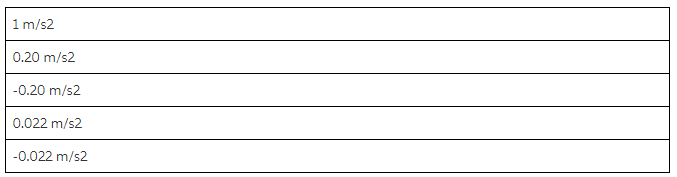Which of the graphs shown below is the correct acceleration vs. time plot for the motion described in the previous parts?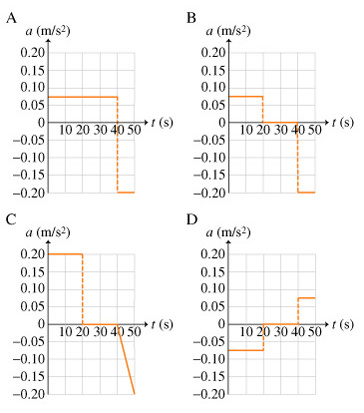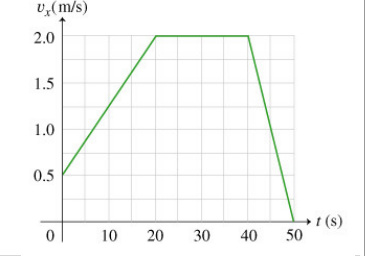Is the above v vs t diagram as as per your question?
If yes, then ans are as follows?

a) Initial speed i.e. at t=0, v=0.5 m/s
b)Total distance travelled =Area of the region under the v vs. t curve

=the distance traveled in the first 20.0 seconds +the distance traveled in the second 20.0 seconds +the distance traveled in the last 10.0 seconds

=25+40+10

Total distance=75m

c) In a v vs. t graph, then, the average acceleration equals the slope of the line connecting the two points representing the initial and final velocities.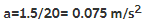d) The instantaneous acceleration of a particle at any point on a v vs. t graph is the slope of the line tangent to the curve at that point.

As it is straight line, acceleration is constant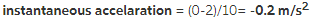e) For graph of a vs t graph, finding the accelation for first 20 sec, second 20 sec and then last -10 sec
so , option B is correct.